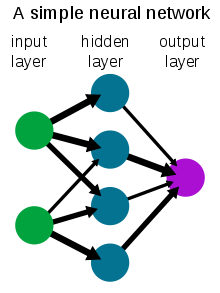## Exploring the Mechanics of Language Models: Understanding Neural Networks and Their Functioning

#### Artificial Intelligence (AI) is a rapidly evolving field that uses computer programming and mathematical algorithms to enable machines to learn and comprehend. AI Language models are a prominent application of this technology, utilizing a neural networks architecture to process data and generate language. .

This paper will provide an overview of AI language models, explaining their technical functioning, the role of neural networks in their functioning, and a brief overview of how neural networks operate.

At a high level, AI language models work by processing language input from a user, then generating an output based on the language models interpretation of that input. The technical process that occurs between a user’s prompt and the AI’s output is usually hidden from the user. Technically, when the user provides an input, that input gets tokenized and fed to the neural network, you can think of this as storing the input as a variable, which subsequently gets passed into the function of the neural network.

Language neural networks are “trained” on vast quantities of data and text (What is a Neural Network? AI and ML Guide – AWS, n.d.). During the training, the network learns to recognize patterns in the data and build a representation of language that can be used to generate responses to the user input. When the users tokenized, input gets fed to the network, the network processes it and extracts relevant information and responds to the user with it in a contextually appropriate form, uses the patterns from its training to understand context.

Many language models also utilize a form of learning called “reinforcement learning” where the model starts to provide more contextually accurate and higher quality information based on user interactions and input, allowing it to improve itself (How Artificial Intelligence Works, n.d.).

A neural network is a collection of interconnected “neurons”, commonly referred to as nodes in the network. In AI systems, the neurons consist of mathematical functions that take in one or more input values, performs a computation on those inputs, and produce an outcome (What is a Neural Network? AI and ML Guide – AWS, n.d.).

The nodes in the network are assembled and interact with each other based on “weights”. Essentially if a certain pattern is more excitatory than another, that node in the network is given a higher weight which feeds it to the next node in the network. After the appropriate nodes have passed through the network, the data reaches the output layer, where the neural networks response gets outputted.

A neural network’s neuron consists of the input values, weights given to the input which determines the strength of the connection between the input and a node in the network, an additional value added to the weighted sum of the inputs known as bias, the activation function which is applied to the weighted sum of the inputs to produce the neurons output, and the output of the neuron which is the result of applying the previously mentioned components. When many neurons are connected in layers, a neural network can learn to recognize patterns and make predictions based on the input data (What is a Neural Network? AI and ML Guide – AWS, n.d.).

The different components of the neurons, or nodes, in the network are adjusted during the training period of the model to optimize the neural network’s performance at a specific task, such as language processing. For example, if you wanted a language model to have certain context, like being limited to certain historical events and language from a certain period, you would train the model based on the information relevant to that time. Below is an image graphic which shows a basic neural network structure. In it the green circles are the inputted values, which get contextually matched up based on the networks training with the appropriate neurons shown by the blue circles, and after the appropriate computation has outputted shown by the purple circle.Source: https://en.wikipedia.org/wiki/Neural_network

In the case of a language AI model, the simplest way to interpret the mechanism is as follows: User inputs data, the neural network then parses the tokenized form of the data and passes it through the network where weights and biases are assigned to various possible outputs, the best fitting output is then selected and presented to the user through the interface on screen. AI language models function in a similar way to the human brain, with the key differences being how the computation is done. The human brain is far more complex than a neural network and is better at learning with very little information that a neural network. Human brains are also highly adaptable, while neural networks can only utilize their training and contextual understanding. Neural networks also lack critical thinking, and language AI neural networks have limited spatial understanding.

The mechanism of AI language models and neural networks is a fascinating topic that has revolutionized the field of computing (How Artificial Intelligence Works, n.d.). By using mathematical algorithms and training on large amounts of data, language models can understand natural language and generate contextually appropriate responses. This is made possible by neural networks which consist of interconnected nodes and can recognize patterns and make predictions based on input data. While neural networks and the human brain have some similarities in their functioning, neural networks are limited in adaptability and critical thinking. However neural network and language AI models have the ability to disrupt many industries and areas of modern life with better and better models likely to arise in the near future.